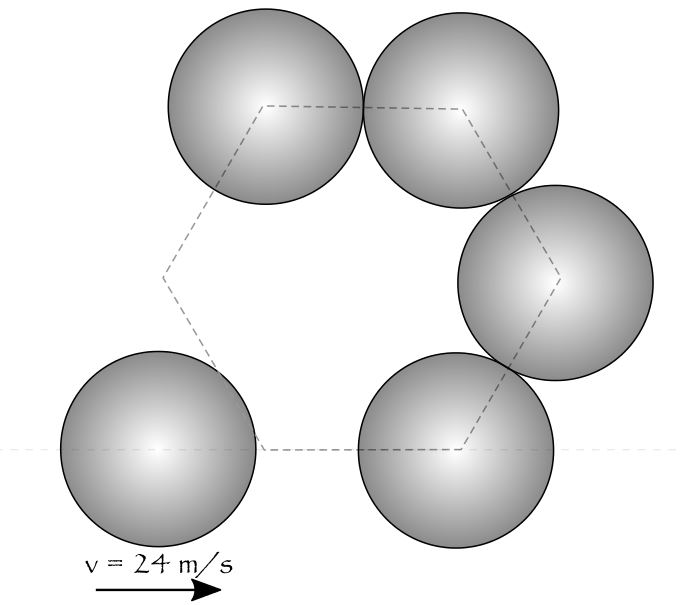Five coins on a tableOf five identical coins (they are circular), four are placed on vertices of a regular hexagon in above fashion. Left one moves with horizontal velocity $24\text{ ms}^{-1}$, to collide with coin on bottom edge along the line joining their centers.

Find the magnitude of velocity with which the coin on top-left edge leaves the hexagon (horizontal velocity, to be precise*).

$\textbf{Details and Assumptions}$

• Consider all surfaces to be smooth.

• Consider all collisions to be elastic.

• Answer in SI Units.

×